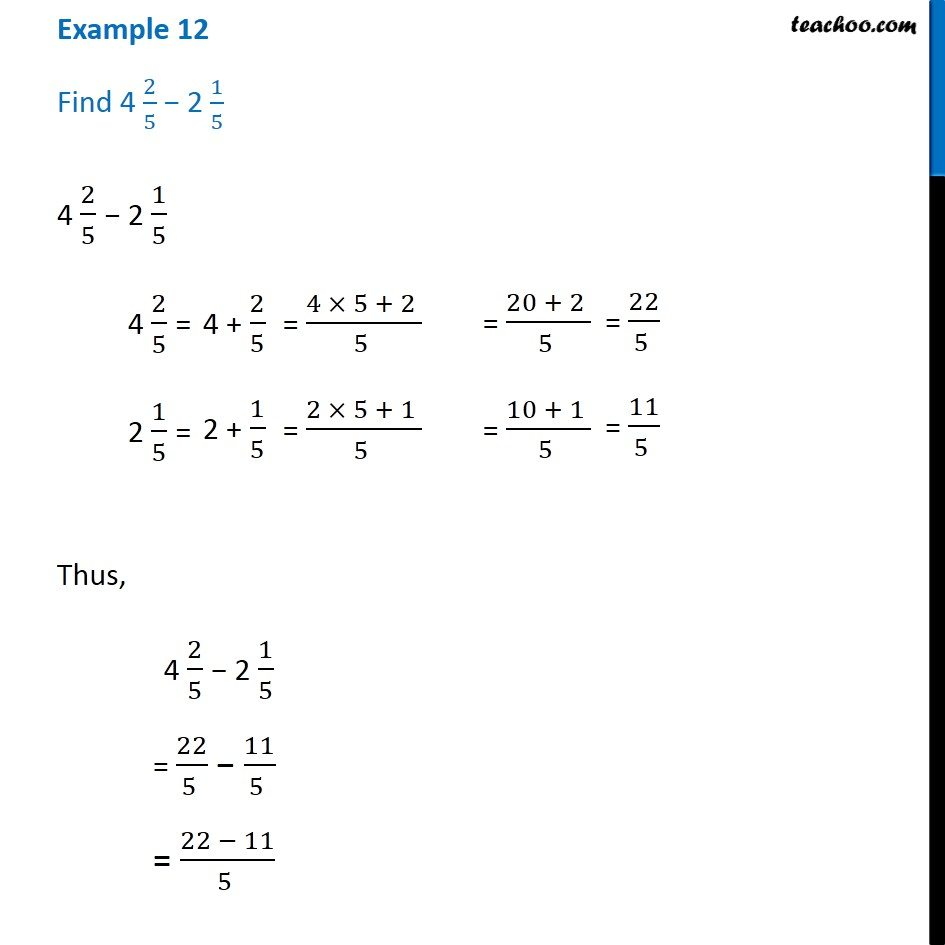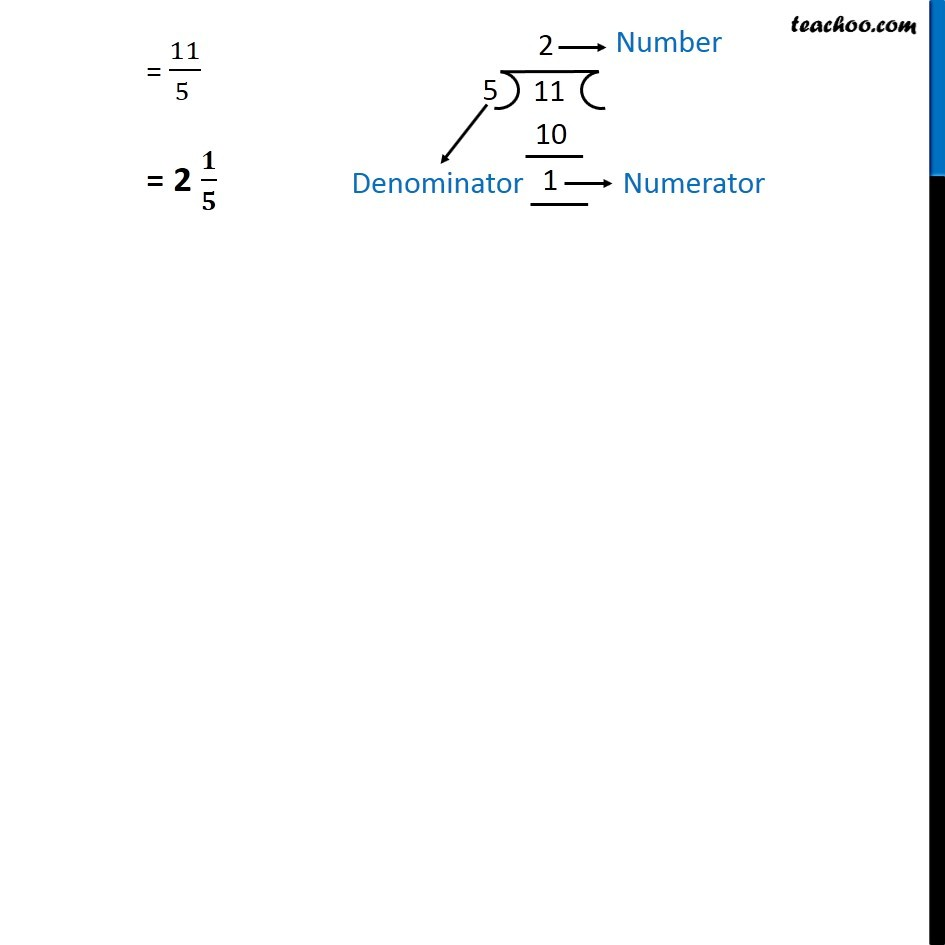Examples

Chapter 7 Class 6 Fractions
Serial order wiseLearn in your speed, with individual attention - Teachoo Maths 1-on-1 Class

### Transcript

Example 12 - Chapter 7 Class 6 Find 4 2/5 − 2 1/5 4 2/5 − 2 1/5 4 2/5 = 4 + 2/5 = (4 x 5 + 2) / 5 = (20 + 2) / 5 = 22/5 2 1/5 = 2 + 1/5 = (2 x 5 + 1) / 5 = (10 + 1) / 5 = 11/5 Thus, 4 2/5 − 2 1/5 = 22/5 − 11/5 = (22 −11)/5 = 11/5 Converting to mixed = 2 1/5